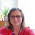## Monday, July 21, 2014

### The Magnified Inch for Fractions

I heard about the Magnified Inch lesson from a colleague about ten years ago and then read about it in a book called Family Math.  The authors put this lesson in the measurement category, but I find it helpful when teaching fractions.  Here is my version of the lesson:

I start by cutting an eighteen inch strip of construction paper for each student.  ( I always make extras.)  I have students fold the ends together to make half.  I label it with a fairly long line, but I leave enough room to show equivalent fractions.

Next, I have the students fold each end to the center.  We talk about cutting each half into half.  I label each part with a slightly shorter line like you would see on a ruler and mark the lines 1/4 and 3/4 respectively.  I tell them that 2/4 is the same thing as 1/2 and write in that label as well.

The next part is where I lose some students.  I need to fold each fourth into half.  I have found that the simplest way to do this is to fold each end of the strip to the 1/4 mark and then to the 3/4 mark.  I label the new marks 1/8, 3/8, 5/8 and 7/8.  I explain that 2/8 = 1/4, 4/8= 2/4=1/2, and 6/8 = 3/4.  Decide how much guidance your students need with labeling equivalent fractions based on how much experience they have had.

I use this tool as a number line.  We talk about which fractions are greater or less than others.  I let them use it as a tool as we go through the unit. I find that because my students have created this tool, they understand it better than a number line printed in a book.

﻿
﻿
﻿

1.I do this :-) not exactly the same way but I do it.

1.I know there are other versions of this activity. What do you do differently? I'd love to know.

2.As important as the number line is, another powerful way for students to better understand (the area model) of equivalent fractions, is to give each student or partner group a piece of standard paper from the photocopier (great way to recycle paper that is no longer needed) and ask the class to fold it in 'half'. What will likely happen is students will either fold it lengthwise or widthwise and both sets of students will declare that they have folded it in 'half'. Stop here and ask student to 'prove' that their 'half' is correct, or 'prove' that the two 'halves', those formed by folding the paper lengthwise and those folding the paper widthwise are equivalent. After moderating the lengthy discussions that will follow from this, students can continue to fold the paper in quarters, eighths, etc, colouring the areas as they go, and they will be very attuned now to noting equivalencies.
In this way, we as teachers, avoid 'telling' students and instead 'guiding' students to understand fractional concepts through these explorations.

1.That's a good idea. I find my students need guiding and telling at different times.